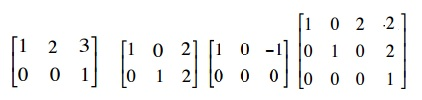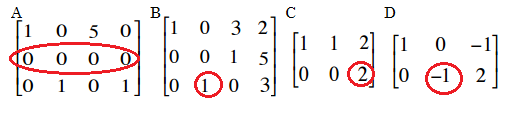# Row Echelon Form & Reduced Row Echelon Form

Watch the video for the definitions of echelon, row echelon and reduced row echelon:

## What is Echelon Form?

Echelon form means that the matrix is in one of two states:

• Row echelon form.
• Reduced row echelon form.

This means that the matrix meets the following three requirements:

1. The first number in the row (called a leading coefficient) is 1. Note: some authors don’t require that the leading coefficient is a 1; it could be any number. You may want to check with your instructor to see which version of this rule they are adhering to).
2. Every leading 1 is to the right of the one above it.
3. Any non-zero rows are always above rows with all zeros.

The following examples are of matrices in echelon form:The following examples are not in echelon form:Matrix A does not have all-zero rows below non-zero rows.
Matrix B has a 1 in the 2nd position on the third row. For row echelon form, it needs to be to the right of the leading coefficient above it. In other words, it should be in the fourth position in place of the 3.
Matrix C has a 2 as a leading coefficient instead of a 1.
Matrix D has a -1 as a leading coefficient instead of a 1.

Another way to think of a matrix in echelon form is that the matrix has undergone Gaussian elimination, which is a series of row operations.

## Uniqueness and Echelon Forms

The echelon form of a matrix isn’t unique, which means there are infinite answers possible when you perform row reduction. Reduced row echelon form is at the other end of the spectrum; it is unique, which means row-reduction on a matrix will produce the same answer no matter how you perform the same row operations.

## What is Row Echelon Form?

A matrix is in row echelon form if it meets the following requirements:

• The first non-zero number from the left (the “leading coefficient“) is always to the right of the first non-zero number in the row above.
• Rows consisting of all zeros are at the bottom of the matrix.

Technically, the leading coefficient can be any number. However, the majority of Linear Algebra textbooks do state that the leading coefficient must be the number 1. To add to the confusion, some definitions of row echelon form state that there must be zeros both above and below the leading coefficient. It’s therefore best to follow the definition given in the textbook you’re following (or the one given to you by your professor). If you’re unsure (i.e. it’s Sunday, your homework is due and you can’t get hold of your professor), it safest to use 1 as the leading coefficient in each row.

If the leading coefficient in each row is the only non-zero number in that column, the matrix is said to be in reduced row echelon form.

Row echelon forms are commonly encountered in linear algebra, when you’ll sometimes be asked to convert a matrix into this form. The row echelon form can help you to see what a matrix represents and is also an important step to solving systems of linear equations.

## Online Row Echelon Form Calculator

This online calculator will convert any matrix, and provides the row operations that get you from step to step. The following image (from the Old Dominion University Calculator shows how the matrix [01, 00, 59] is reduced to row echelon form with two simple row operations:## What is Reduced Row Echelon Form?

Reduced row echelon form is a type of matrix used to solve systems of linear equations. Reduced row echelon form has four requirements:

• The first non-zero number in the first row (the leading entry) is the number 1.
• The second row also starts with the number 1, which is further to the right than the leading entry in the first row. For every subsequent row, the number 1 must be further to the right.
• The leading entry in each row must be the only non-zero number in its column.
• Any non-zero rows are placed at the bottom of the matrix.

## Transformation of a Matrix to Reduced Row Echelon Form

Any matrix can be transformed to reduced row echelon form, using a technique called Gaussian elimination. This is particularly useful for solving systems of linear equations. Most graphing calculators (like the TI-83) have a rref function which will transform a matrix into a reduced row echelon form. See: This article on the Colorado State University website for instructions on using the TI-89 and TI-83 to calculate reduced row echelon form.
This online calculator on the Old Dominion University website transforms a matrix that you input to reduced row echelon form.

Calculation by hand requires knowledge of elementary row operations, which are:

1. Interchange one row with another.
2. Multiply one row by a non-zero constant.
3. Replace one row with: one row, plus a constant, times another row.

In addition, it isn’t enough just to know the rules, you have to be able to look at the matrix and make a logical decision about which rule you’re going to use and when. You’re trying to get the matrix into reduced row echelon form, so you’ll also need to refer to the four requirements at the beginning of this article. If you have to convert a matrix to reduced row echelon form by hand, it’s a good idea to use one of the calculators above to check your work. In fact, of you use the ODU online calculator, it will even provide the row operations for you. The image below is the calculator’s conversion of the matrix [204,923]:## What is Gaussian Elimination?

Gaussian elimination is a way to find a solution to a system of linear equations. The basic idea is that you perform a mathematical operation on a row and continue until only one variable is left. For example, some possible row operations are:

• Interchange any two rows
• Multiply one row by a non-zero constant (i.e. 1/3, -1, 5)

You can also perform more than one row operation at a time. For example, multiply one row by a constant and then add the result to the other row.

Following this, the goal is to end up with a matrix in reduced row echelon form where the leading coefficient, a 1, in each row is to the right of the leading coefficient in the row above it. In other words, you need to get a 1 in the upper left corner of the matrix. The next row should have a 0 in position 1 and a 1 in position 2. This gives you the solution to the system of linear equations.

## Gaussian Elimination Example

Solve the following system of linear equations using Gaussian elimination:

• x + 5y = 7
• -2x – 7y = -5

Step 1: Convert the equation into coefficient matrix form. In other words, just take the coefficient for the numbers and forget the variables for now:Step 2: Turn the numbers in the bottom row into positive by adding 2 times the first row:Step 3: Multiply the second row by 1/3. This gives you your second leading 1:Step 4: Multiply row 2 by -5, and then add this to row 1:That’s it!
In the first row, you have x = -8 and in the second row, y = 3. Note that x and y are in the same positions as when you converted the equation in step 1, so all you have to do is read the solution:## What is the Rank of a Matrix?

The rank of a matrix is equal to the number of linearly independent rows. A linearly independent row is one that isn’t a combination of other rows.

The following matrix has two linearly independent rows (1 and 2). However, when the third row is thrown into the mix, you can see that the first row is now equal to the sum of the second and third rows. Therefore, the rank of this particular matrix is 2, as there are only two linearly independent rows.The matrix rank will always be less than the number of non-zero rows or the number of columns in the matrix. If all of the rows in a matrix are linearly independent, the matrix is full row rank. For a square matrix, it is only full rank if its determinant is non-zero.

Figuring out the rank of a matrix by trying to determine by sight only how many rows or columns are linearly independent can be practically impossible. An easier (and perhaps obvious) way is to convert to row echelon form.

## How to Find the Matrix Rank

Finding the rank of a matrix is simple if you know how to find the row echelon matrix. To find the rank of any matrix:

1. Find the row echelon matrix.
2. Count the number of non-zero rows.

The above matrix has been converted to row echelon form with two non-zero rows. Therefore, the rank of the matrix is 2.

You can also find an excellent conversion tool on the Old Dominion University website.